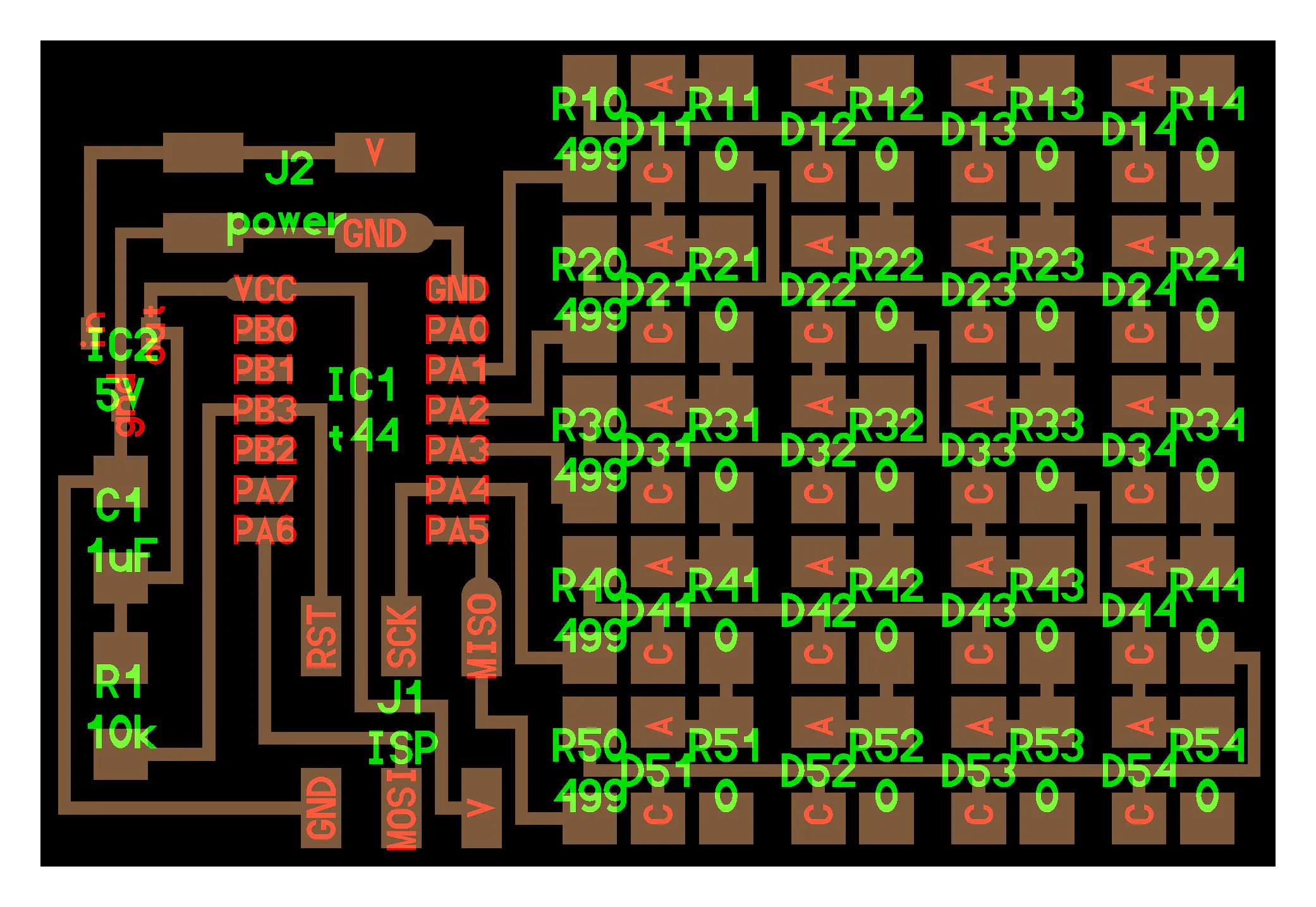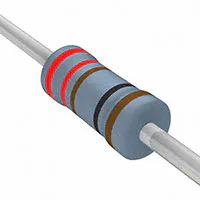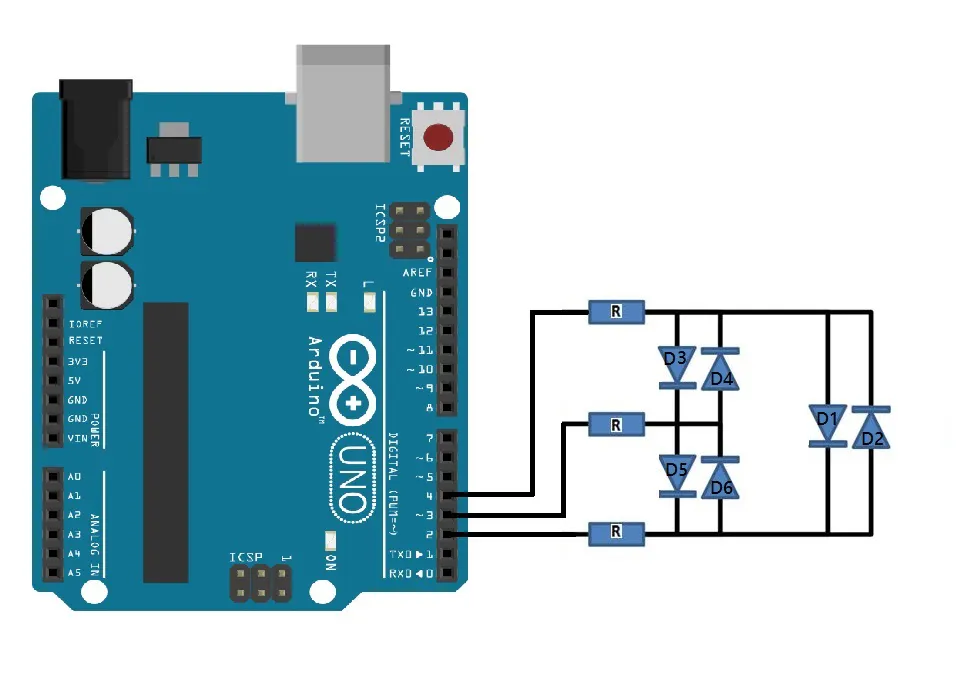# Charlieplexing with Arduino

Charlieplexing extends the limitation of I/O pins of a controller beyond its capability.

EasyFull instructions provided1 hour3,360## Things used in this project

### Hardware componentsArduino UNO & Genuino UNO
×1Resistor 221 ohm
×1LED (generic)
×1

### Hand tools and fabrication machines

 Arduino CC

## Schematics

### Schematic Diagram## Code

### Arduino Code for Charlieplexing

C/C++
```int A = 2;
int B = 3;
int C = 4;

void setup()
{
pinMode(A, OUTPUT);
pinMode(B, OUTPUT);
pinMode(C, OUTPUT);

}

/*
* Turn on the given LED
*
* @paramledNum LED to turn on (1..6)
*
*/
void setLED(int ledNum)
{
if(ledNum == 1)
{
pinMode(A, OUTPUT);
pinMode(B, INPUT);  // changed to input to trigger tri state.
pinMode(C, OUTPUT);

digitalWrite(A, HIGH);
digitalWrite(C, LOW);
}

if(ledNum == 2)
{
pinMode(A, OUTPUT);
pinMode(B, INPUT);
pinMode(C, OUTPUT);

digitalWrite(A, LOW);
digitalWrite(C, HIGH);
}

if(ledNum == 3)
{
pinMode(A, OUTPUT);
pinMode(B, OUTPUT);
pinMode(C, INPUT);

digitalWrite(A, HIGH);
digitalWrite(B, LOW);
}

if(ledNum == 4)
{
pinMode(A, OUTPUT);
pinMode(B, OUTPUT);
pinMode(C, INPUT);

digitalWrite(A, LOW);
digitalWrite(B,HIGH);
}

if(ledNum == 5)
{

pinMode(A, INPUT);
pinMode(B, OUTPUT);
pinMode(C, OUTPUT);

digitalWrite(B, HIGH);
digitalWrite(C, LOW);
}

if(ledNum == 6)
{

pinMode(A, INPUT);
pinMode(B, OUTPUT);
pinMode(C, OUTPUT);

digitalWrite(B, LOW);
digitalWrite(C, HIGH);
}
}

void loop()
{

for(;;)
{
setLED(1);
delay(500);
setLED(2);
delay(500);
setLED(3);
delay(500);
setLED(4);
delay(500);
setLED(5);
delay(500);
setLED(6);
delay(500);
}

}
```

## Credits

### Lilanka Udawatta

6 projects • 33 followers
I am Electrical and Electronic engineering student, seeking for opportunities to create something that actually matter to the society.